# Objective 10. Design a Worksheet for What-If Analysis

Excel recalculates; if you change the value in a cell referenced in a formula, the result of the formula is automatically recalculated. Thus, you can change cell values to see what would happen if you tried different values. This process of changing the values in cells to see how those changes affect the outcome of formulas on the worksheet is called What-if analysis.

Activity 2.17. Calculating a Percentage Rate of Increase

Ms. French has the city's population figures for the first year of each decade for the past five decades. Each decade, the population has increased. In this activity, you will build a formula to calculate the percentage rate of increasethe percent by which one number increases over another numberfor each decade over the past 50 years. From this information, future population growth can be estimated.

1.

Start Excel and Close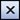the Getting Started task pane. From the File menu, click Save As. In the Save As dialog box, navigate to the drive and folder where you are storing your projects for this chapter. In the File name box, type 2C_Population_Growth_Firstname_Lastname and then click Save or press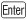.

2.

In cell A3 type Population and press. In cell A4 type Percent Increase and press. Select column A. In the column heading area, point to the right boundary of column A to display the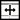pointer, and then double-click to AutoFit the column to accommodate its longest entry. Alternatively, from the Format menu, point to Column, and then click AutoFit Selection.

3.

In cell A2, type Decade In cell B2, type 1960 and press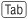. In cell C2 type 1970 and press. Select the range B2:C2, drag the fill handle to the right through cell F2, and then compare your screen with Figure 2.42.

Figure 2.42.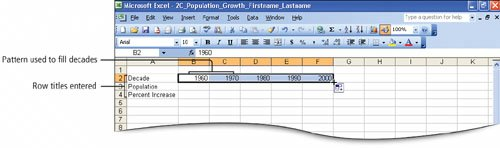By establishing a pattern of 10-year intervals with the first two cells, you can use the fill handle to continue the series.

4.

Click cell A1. Type Past Population and press. Select the range A1:F1, click the Merge and Center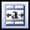button, change the Font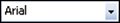to Arial Black and the Font Size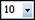to 18.

5.

Beginning in cell B3 and pressingto move across the row, enter the following values for the population in the years listed:

 1960 1970 1980 1990 2000 307419 409012 552793 697127 945302

6.

Select the range B3:F3, apply the Comma Style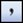, and then click Decrease Decimaltwo times.

7.

Click cell C4. Type =(c3-b3)/b3 and press. Click cell C4, click the Percent Style button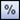, and then look at the formula in the Formula Bar.

The mathematical formula to calculate the percentage rate of increase from 1960 to 1970 is rate = amount of increase/base.

The first step is to determine the amount of increase. This is accomplished by subtracting the basethe starting pointrepresented by the 1960 population, from the 1970 population. Thus, the amount of increase = 409,012 307,419 or 101,593. Between 1960 and 1970, the population increased by 101,593 people. In the formula, this calculation is represented by c3-b3.

The second step is to calculate the ratewhat the amount of increase (101,593) represents as a percentage of the base (1960's population). Determine this by dividing the amount of increase (101,593) by the base (307,419). Thus, 101,593 divided by 307,419 is equal to 0.330471 or, when rounded to a percent33%.

8.

In the Formula Bar, notice the parentheses enclosing C3-B3.

NoteExcel follows a mathematical rule called the order of operations, which has four basic parts:

• Expressions within parentheses are processed first.
• Exponentiation, if present, is performed before multiplication and division.
• Multiplication and division are performed before addition and subtraction.
• Consecutive operators with the same level of precedence are calculated from left to right.

9.

Click cell D4, press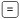, and then by either typing or clicking cells, construct a formula similar to the one in cell C4 to calculate the rate of increase in population from 1970 to 1980. Then, compare your screen with Figure 2.43.

Figure 2.43.

(This item is displayed on page 740 in the print version)Recall that the first step is to determine the amount of increase1980 population minus 1970 populationand then to write the calculation so that Excel performs this operation first; that is, place it in parentheses.

The second step is to divide the result of the calculation in parentheses by the basethe population for 1970.

10.

Pressand then format cell D4 with the Percent Style. Your result is 35%.

11.

With cell D4 selected, drag the fill handle to the right through cell F4. Click any empty cell to cancel the selection, and then compare your screen with Figure 2.44.

Figure 2.44.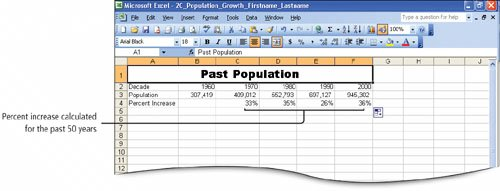Because this formula uses relative cell referencesthat is, for each year, the formula is the same but the values used are relative to the formula's locationyou can copy the formula in this manner. For example, the result for 1980 uses 1970 as the base, the result for 1990 uses 1980 as the base, and the result for 2000 uses 1990 as the base.

The formula results show the percent of increase for each decade between 1960 and 2000. You can see that each decade, the population has grown as much as 36%between 1990 and 2000and as little as 26%between 1980 and 1990.

More Knowledge: Use of Parentheses in a Formula

When writing a formula in Excel, parentheses are used to communicate the order in which the operations should occur. For example, to average the grades you have received in a class, you would add the grades and then divide by the number of grades in the list. If you write this formula as =100+50+90/3, the results would be 180, because Excel would first divide 90 by 3 and then add 100+50+30. The correct way to write this formula is =(100+50+90)/3. In this example, Excel would add the three values, and then divide the result by 3, or 240/3 resulting in a correct average of 80. Parentheses play an important role in assuring that you get the correct results in your formulas.

Activity 2.18. Formatting as You Type

You can format numbers as you type them. When you type numbers in a format that Excel recognizes, Excel automatically applies that format to the cell. Recall that once applied, cell formats remain with the cell, even if the cell contents are deleted. In this activity, you will format cells by typing the numbers with percent signs and you will use the Format Painter to copy text (non-numeric) formats.

 1. In cell A6 type Projected Population and press. Click cell A1. On the Standard toolbar, click the Format Painter button, and then click cell A6. The format that is applied to cell A1 is painted or applied to cell A6, including the merging and centering of the text across cells A6:F6. 2. In cell A8 type Estimated Growth Rate and then press. AutoFit column A to accommodate the new longer entry. 3. In cell A10 type Decade and in cell A11 type Projected Population In cell B10 type 2000 and then press. In cell C10 type 2010 Select the range B10:C10 and then drag the fill handle through cell F10 to extend the pattern of years to 2040. Compare your screen with Figure 2.45.     Figure 2.45.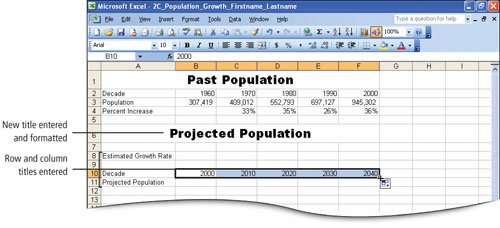4. In cell B11, type 945,302 and on the Formula Bar click the Enter button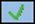so that the cell remains selected. Then, pressand without typing a comma, type 945302 and press. The comma is inserted even though you did not type it. When you type a number and include a formatting symbol such as a comma, dollar sign, or percent sign, Excel applies the format to the cell. Thus, if you delete the contents of the cell and type in the cell again, the format you established remains applied to the cell. This is referred to as format as you type. 5. Examine the format of the value in cell B11 and compare it to the format in cell B3 where you used the Comma Style button to format the cell. Notice that the number in cell B11 is flush with the right edge of the cell, but the number in cell B3 leaves a small space on the right edge. When you type commas as you enter numbers, Excel applies the Number format, which does not leave a space at the right of the number for a closing parenthesis in the event of a negative number. This is different from the format that is applied when you use the Comma Style button on the Formatting toolbar, as you did for the numbers entered in row 3. The Comma Style buttons apply the Accounting format, but without the dollar sign. The Accounting format leaves space on the right for a closing parenthesis. 6. In cell B8, type 26% and on the Formula Bar click Enter. Then, pressand without typing a percent sign, type 26 and press. The percent sign is inserted even though you did not type itanother example of the format as you type feature. 7. Save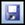your workbook.

More Knowledge: Percentage Calculations

When you type a percentage into a cell, for example 26%, the percentage format, without decimal points, displays in both the cell and the Formula Bar. However, Excel will use the 0.26 decimal value for actual calculations.

Activity 2.19. Calculating a Value After an Increase

A growing population results in increased use of streets, schools, and other city services. Thus, city planners in Desert Park must estimate how much the population will increase in the future. The calculations you made in the previous activity show that the population has increased at varying rates each decade, ranging from a low of 26% to a high of 36% per decade.

To plan for the future, Ms. French wants to prepare three forecasts of the city's population based on the lowest, highest, and average growth rates. In this activity, you will calculate the population increases that would result for the lowest rate of growth.

 1. Click cell C11. Type =b11*(100%+\$b\$8) and then on the Formula Bar click Enter. Compare your screen with Figure 2.46.   Figure 2.46. (This item is displayed on page 744 in the print version)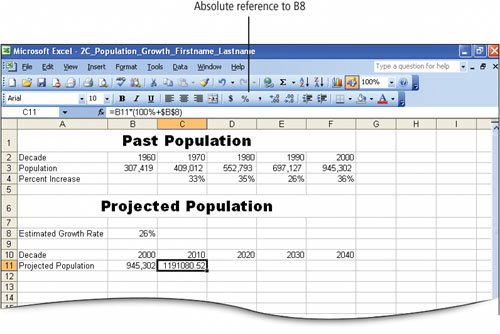This formula calculates what the population will be in the year 2010 assuming an increase of 26% over 2000's population. The mathematical formula to calculate a value after an increase is value after increase = base x percent for new value. The first step is to establish the percent for new value. The percent for new value = base percent + percent of increase. The base percent of 100% represents the base population and the percent of increase in this instance is 26%. Thus, the population will equal 100% of the base year plus 26% of the base year. This can be expressed as 126% or 1.26. In this formula, you will use 100% + the rate in cell B8 to equal 126%. The second step is to enter a reference to the cell that contains the basethe population in 2000. This value resides in cell B11945,302. The third step is to calculate the value after increase. Because each decade's increase will be based on 26%an absolute value located in B8this cell reference can be formatted as absolute with the use of dollar signs. 2. With cell C11 as the active cell, drag the fill handle to fill the formula into D11:F11. Click cell B11, click Format Painter, and then select the range C11:F11. Click an empty cell to deselect, and then compare your screen with Figure 2.47.   Figure 2.47. (This item is displayed on page 745 in the print version)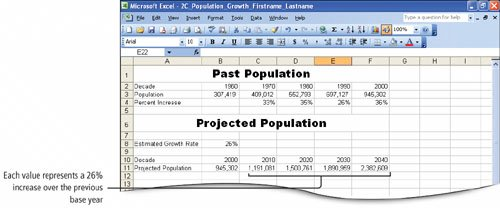This formula uses a relative cell addressB11for the base; the population in the previous decade is used in each of the formulas in cells D11:F11 as the base value. Because the reference to the percent of increase in cell B8 is an absolute reference, each value after increase is calculated with the value from cell B8. The population projected for 20101,191,081is an increase of 26% over the population in 2000. The population in 20201,500,761is an increase of 26% over the population in 2010 and so on. 3. Saveyour workbook.

More Knowledge: Calculating Percent Increase or Decrease

The basic formula for calculating an increase or decrease can be done in two parts. First determine the percent by which the base value will be increased or decreased, and then add or subtract the results to the base. The formula can be simplified by using (1+Amount of increase) or (1-Amount of decrease), where 1 represents the whole, rather than 100%. So the formula used in step 1 could also be written =b11*(1+\$b\$8), or =(b11*\$b\$8)+b11.

[Page 745 (continued)]

### Objective 11 Perform What If Analysis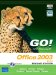GO! with Microsoft Office 2003 Brief (2nd Edition)
ISBN: 0131878646
EAN: 2147483647
Year: 2004
Pages: 448